s-t Flow

Capacity Graph（Flow Network）「容量圖」只有容量資訊，沒有流量資訊。

s-t Flow

s-t Flow以下簡稱為「流」。一個流便是由源點經管線至匯點的水流。一個流的流量，即是源點灌入的水流流量，同時也是匯點泄出的水流流量。

「流」只有流量資訊，沒有容量資訊。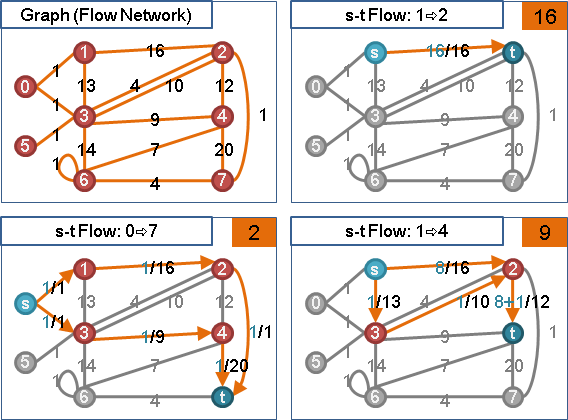Maximum s-t Flow

「最大流」。給定一張圖，以及給定一個源點與一個匯點，所有可能的s-t Flow當中，流量最大者便是Maximum s-t Flow，可能會有許多個。Minimum s-t Flow

「最小流」。一滴水都不流，管線裡都沒水，流量為零。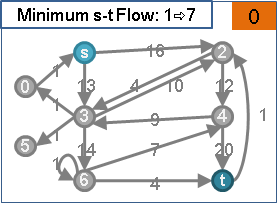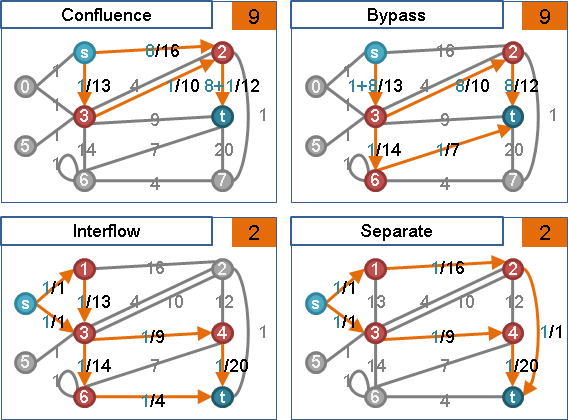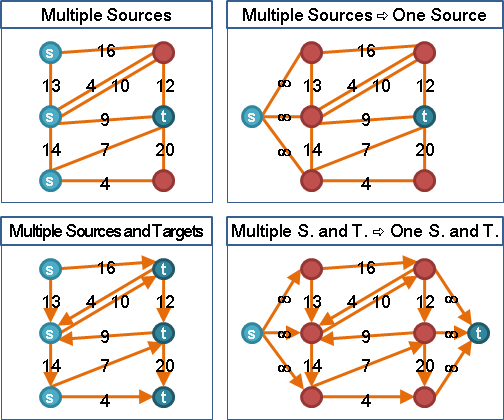Max-Flow Min-Cut Theorem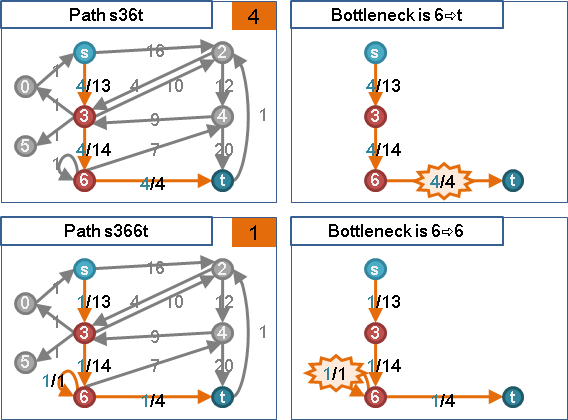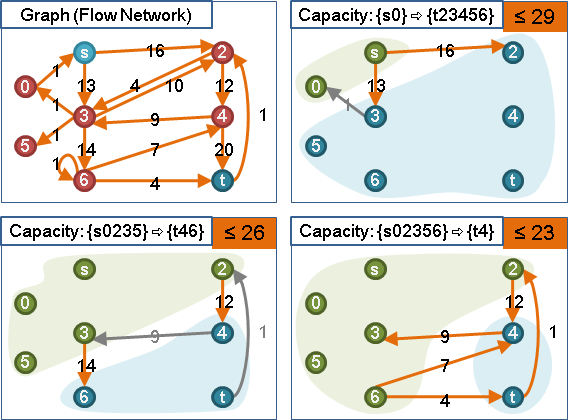【關於s-t Cut，請見本站文件「s-t Cut」。】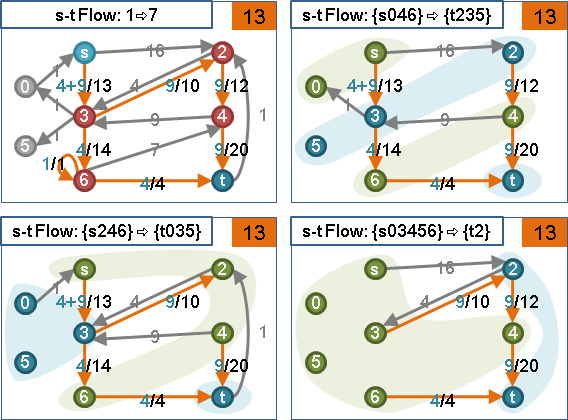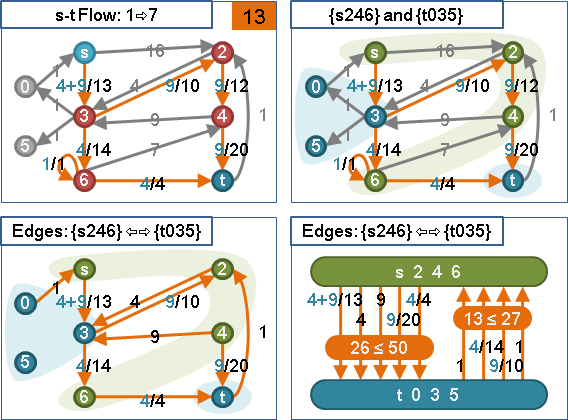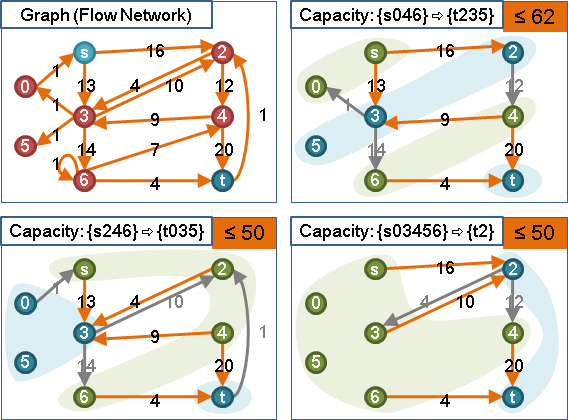Max-Flow Min-Cut Theorem

「最大流最小割定理」。「最大st流」等於「最小st割」。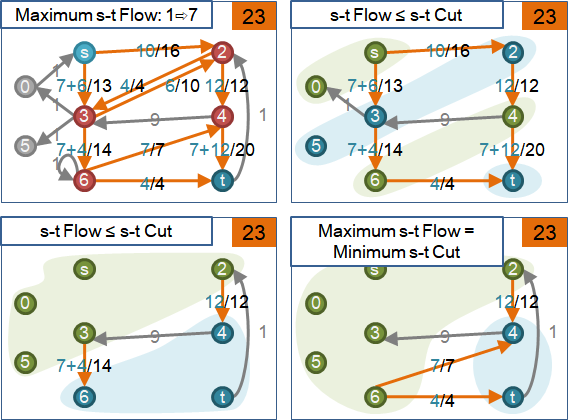Maximum s-t Flow: Ford–Fulkerson Algorithm (Augmenting Path Algorithm)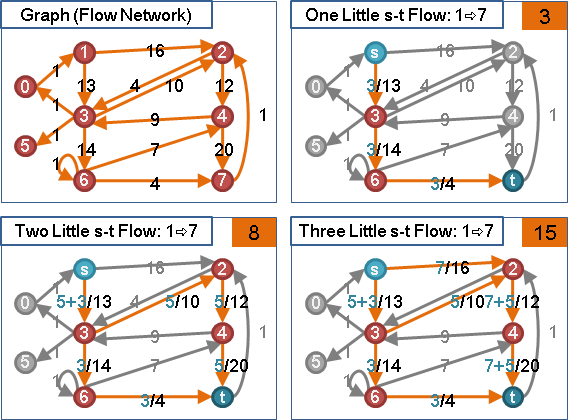【註：涓流、溯洄、沖減這三個詞，是初次使用。我查字典後，覺得意義相近，而選用的。而且它們都是水字旁！】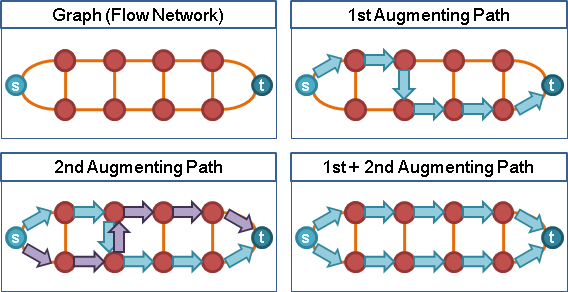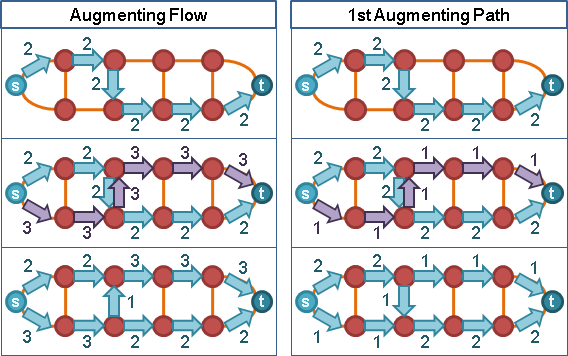UVa 10806 10380

Residual GraphAugmenting Path

「擴充路徑」。剩餘圖上面一條源點到匯點的路徑。把握剩餘容量，把握溯洄沖減，找出一條源點到匯點的涓流路徑，就是一條擴充路徑。```不斷找擴充路徑，並擴充流量。直到沒有擴充路徑為止。
```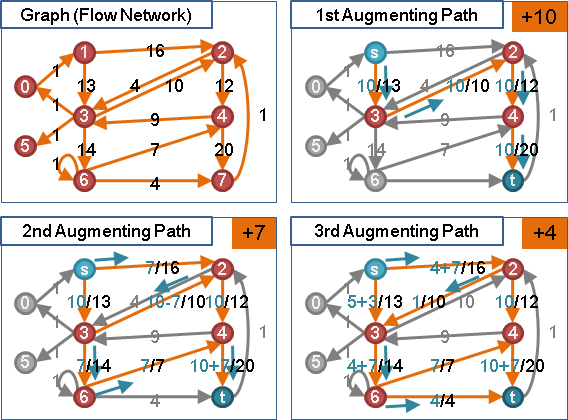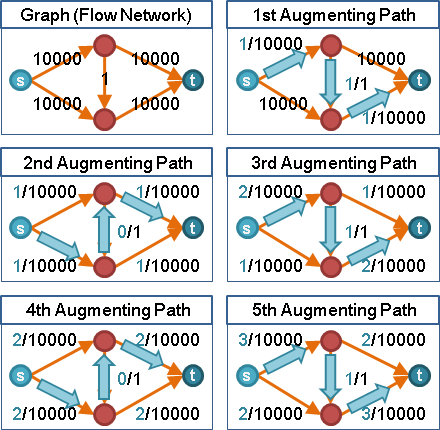```最初沒有水流：
flow(i, j) = 0;
flow(j, i) = 0;

flow(i, j) += 流量;
flow(j, i) 保持不變;

flow(i, j) += 流量1;
flow(j, i) += 流量2;

flow(i, j) += 流量;
flow(j, i) 保持不變;
residual(i, j) = capacity(i, j) - (flow(i, j) - flow(j, i));
residual(j, i) = capacity(j, i) - (flow(j, i) - flow(i, j));
```

```最初沒有水流：
flow(i, j) = 0;
flow(j, i) = 0;

flow(i, j) += 流量;
flow(j, i) -= 流量;

flow(i, j) = flow(i, j) + 流量1 - 流量2;
flow(j, i) = flow(j, i) - 流量1 + 流量2;

flow(i, j) += 流量;
flow(j, i) = -flow(i, j);
residual(i, j) = capacity(i, j) - flow(i, j);
residual(j, i) = capacity(j, i) - flow(j, i);
```

```最初沒有水流：
residual(i, j) = capacity(i, j);
residual(j, i) = capacity(j, i);

residual(i, j) -= 流量;
residual(j, i) += 流量;

residual(i, j) = residual(i, j) - 流量1 + 流量2;
residual(j, i) = residual(j, i) + 流量1 - 流量2;

residual(i, j) -= 流量;
residual(j, i) += 流量;
flow(i, j) = capacity(i, j) - residual(i, j);
flow(j, i) = capacity(j, i) - residual(j, i);
```

Maximum s-t Flow: Edmonds–Karp Algorithm (Shortest Augmenting Path Algorithm)

Augmenting Path Algorithm改良版。擴充路徑是源點到匯點的最短路徑（管線長度皆為1），並且擴充流量至瓶頸。這種方式可以避免浪費管線空間，避免反覆地溯洄沖減，更快找到最大流。

```不斷找最短擴充路徑，直到找不到為止，即得最大流。

```

（Edge Labeling with Shortest Distance）

```瓶頸正向邊：距離數值增加，變成無限大。（水滿了，從剩餘圖之中消失。）

距離數值增加，比正向邊至少多一。（原先沒有反向邊，新增反向邊。）
其餘的邊：距離數值不變。（瓶頸斷掉，但是有替代路線。或者尚未遭遇瓶頸。）
距離數值增加。（瓶頸斷掉，繞遠路。）
```

（Vertex Labeling with Shortest Distance）

UVa 820 10330 10779 563 10511 10983

Maximum s-t Flow: Dinitz' Algorithm (Blocking Flow Algorithm)

Shortest Augmenting Path Algorithm改良版。一口氣找到一樣長的最短擴充路徑們。

```重覆以下動作最多V-1次，直到無法擴充流量：
1. 計算residual graph各點到源點（匯點）的最短距離。
3. 尋找blocking flow，並擴充流量。
```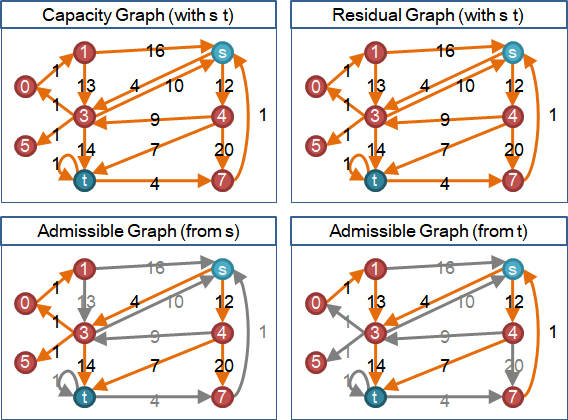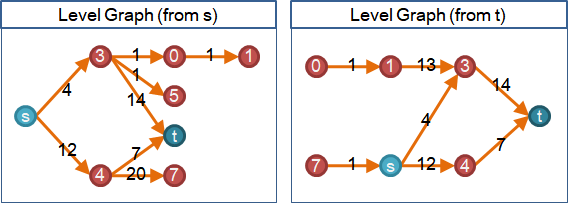Blocking Flow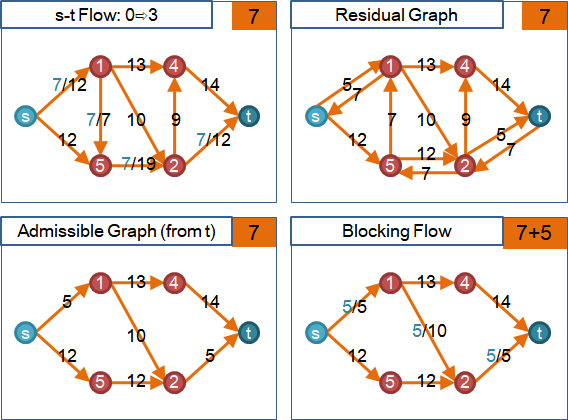（Vertex Labeling with Shortest Distance）

UVa 10546

Maximum s-t Flow: Karzanov's Algorithm (Preflow Algorithm)

《Combinatorial Algorithms: Enlarged Second Edition》。

Blocking Flow Algorithm改良版。只改善阻塞流的演算法。

Backtracking（不是DFS）走訪容許圖，依照拓樸順序。從源到匯，逐邊送水，逐點積水。針對當前點：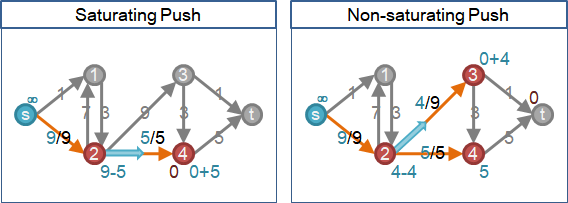Backtracking僅僅調動了送水順序。灌不滿的次數仍然一樣多。

```一、分層圖推廣成有向無環圖。

演算法過程當中，不再處理源點，節省時間。

每個點各自建立queue，儲存出邊剩餘容量，以便前進。
每個點各自建立stack，儲存入邊流量，以便回溯。

積水充足，按照拓樸順序接連前進，直到沒有任何一點能夠前進。
積水過剩，按照拓樸逆序接連回溯，直到沒有任何一點積水過剩。
```
```stack            入邊流量
queue            出邊剩餘容量
push             出邊增加水量
balance          入邊依照LIFO順序減少水量，直到此點出入水量相等
excess           所有入邊水量減掉所有出邊水量
flow             源匯以外每一點出入水量相等
preflow          源匯以外有些點出入水量不相等
blocking flow    源點到匯點的所有路徑都出現瓶頸
blocking preflow 任一點到匯點的任一條路徑出現瓶頸
```

Maximum s-t Flow: Goldberg–Tarjan Algorithm (Push–Relabel Algorithm)

🕛Preflow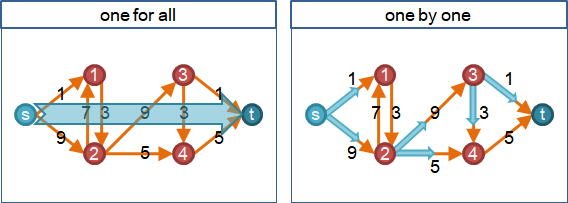🕐Excess

```以「積水點」和「積水量」設計最大流演算法：

```🕑Residual Capacity

```以「剩餘容量」設計最大流演算法：

```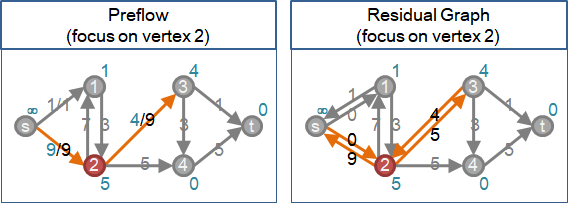🕒Height

```以「水往低處流」設計最大流演算法：

```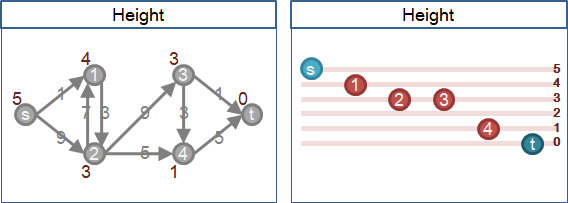```以「一點接著一點慢慢送水」設計最大流演算法：

```🕔Relabel🕕Push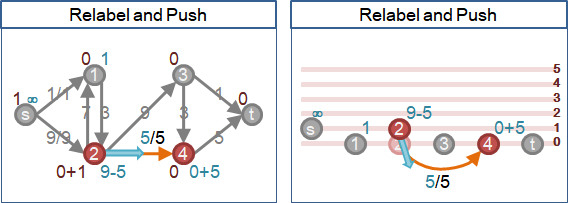🕖Retreat🕗Discharge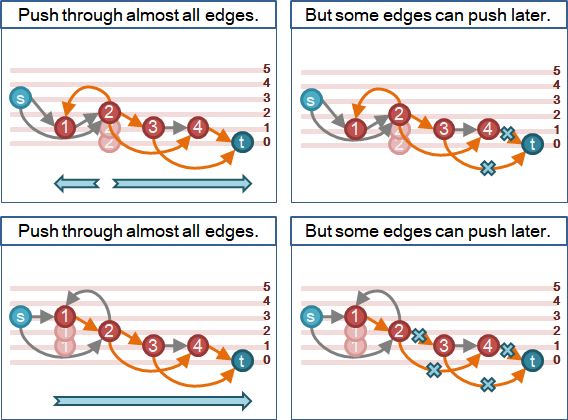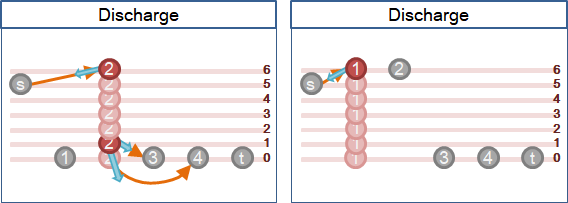🕘Complete Retreat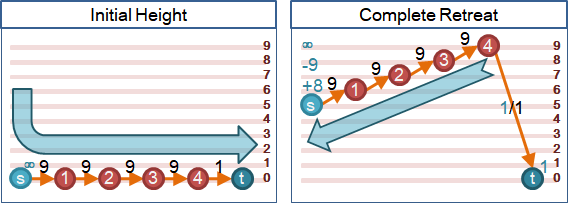🕙Source Discharge🕚Source Height```方才設計的最大流演算法：

```

Push

```一、積水點不是源點和匯點。（源點已排水，匯點只收水。）

```Relabel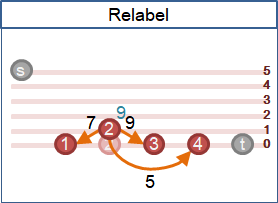Discharge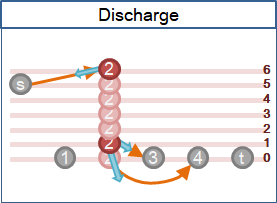HeightPreflow【原始論文將Source Discharge叫做Preflow】（Push–Relabel Algorithm）

```1. height。（可省略）
2. preflow。（可省略，但是要限制源點高度低於V。）
3. 不斷push或relabel，次序隨意，直到無法進行為止。
```

relabel抬高：源點高度設為V-1，匯點高度設為0。最差情況下，除源點匯點，最高點升到了2V-3、最低點升到了V。saturating push灌到滿：一條邊，兩個端點逐漸升高，高度範圍為0到2V-3，高度相差一才能送水，一種高度最多送水一次。non-saturating push灌不滿：同上，但是端點可從鄰點補充水量，最多補充V-2次。一種高度最多送水V-1次。```1. height。（可省略）
2. preflow。（可省略，但是要限制源點高度低於V。）
3. 不斷discharge，次序隨意，直到無法進行為止。
```

（FIFO Push–Relabel Algorithm）

```1. 建立一個queue，塞入積水點（不包括源點匯點），積水點不重複。
2. queue不斷彈出點，進行discharge，直到queue無點。
當discharge產生新的積水點，而且queue沒有這個積水點，就塞入queue。
```

relabel抬高：時間複雜度同前。

saturating push灌到滿：時間複雜度同前。

non-saturating push灌不滿：援引Preflow Algorithm的分析技巧。所有邊O(V³)次。總共O(V³)。

（Highest-Label Push–Relabel Algorithm）

Maximum s-t Flow: Ahuja–Orlin Algorithm (Distance-directed Augmenting Path Algorithm)

《Network Flows: Theory, Algorithms, and Applications》。

（Distance-directed Augmenting Path Algorithm）

Push–Relabel Algorithm改良版。放棄逐點送水，重啟擴充路徑。

DFS走訪容許圖，尋找擴充路徑。若遇到死胡同，就relabel、retreat，另覓路徑。若順利走到匯點，就形成一條擴充路徑，並且擴充流量。

UVa 10983

（Distance-directed Push-Relabel Algorithm）

Preflow Algorithm能夠優先找到每一點到匯點的阻塞流（水足夠時），形成局部的阻塞流！每次回溯皆實施relabel，隨時調校局部的最短路徑長度！

```Ford–Fulkerson Algorithm
Augmenting Path Algorithm

Edmonds–Karp Algorithm
Shortest Augmenting Path Algorithm

Dinitz' Algorithm
Blocking Flow Algorithm

Ahuja–Orlin Algorithm
Distance-directed Augmenting Path Algorithm

```

Maximum s-t Flow: Gabow's Algorithm (Capacity Scaling Algorithm)

```重複以下步驟logC回合：
1. 每條邊容量翻倍：流量隨著翻倍。
2. 每條邊容量加零或加一。
3. 填滿多出的容量，達到最大流。
```

```最大流問題只有四個要素：
甲、容量（Capacity Graph）。甲≥0。
乙、流量（s-t Flow）。甲≥乙≥0。
丙、剩餘容量（Residual Graph）。甲減乙、反向乙。

給定甲暨源點匯點，令乙趨近甲，求乙。

以丙的視角看問題，有隙就流。（最大流最小割定理）
以丁的視角看問題，可以縮短流動路線，加速演算法。

一、擴充路徑（率先流到匯點）
Ford–Fulkerson Algorithm         丙上隨便找一條擴充路徑，不斷找。
Augmenting Path Algorithm        O(EF)

Edmonds–Karp Algorithm           丙上BFS找一條擴充路徑，最多VE次。
Shortest AP Algorithm            O(VEE)

Dinitz' Algorithm                丁上多次DFS找擴充流，最多V-1次。
Blocking Flow Algorithm          O(VVE)

Ahuja–Orlin Algorithm            丁上DFS找擴充路徑，最多VE次。
Distance-directed AP Algorithm   O(VVE)

Gabow's Algorithm                限制甲尺度找擴充流，最多logC次。
Capacity Scaling Algorithm       O(VElogC)

二、預流（率先流離源點）
Preflow Algorithm                          回溯推  O(VVV)
Push–Relabel Algorithm                     隨性推  O(VVE)
FIFO Push–Relabel Algorithm                FIFO推  O(VVV)
Highest-Label Push–Relabel Algorithm       最高推  O(VVsqrtE)
Distance-directed Push–Relabel Algorithm   回溯推  O(VVE)
```